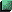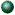# Introduction to Finite Element Method

## Course Numbers: 20-MECH-5025;   Fall Semester

Instructor: Dr. Yijun Liu, Professor of Mechanical EngineeringShowcase: Finite Element Analysis in ActionsClick here to see some examples of the  final projects from this course and other FEA applications in engineeringCourse SyllabusFEM (Fall Semester)Contents of the LecturesChapter 1. Introduction

• Lecture 1. Introduction to FEM
• Lecture 2. Review of Matrix Algebra
• Lecture 3. Stiffness Matrix for Spring Element; FE Equations
• Lecture 4. Assembly of Stiffness Matrices; Examples
• Homework ProblemsChapter 2. Bar and Beam Elements. Linear Static Analysis

• Lecture 1. Linear Static Analysis; Bar Element
• Lecture 2. Examples
• Lecture 3. Distributed Load; Transformation of Coordinate Systems; Element Stress
• Lecture 4. Examples
• Lecture 5. Introduction to ANSYS (Computer Lab Session 1)
• Lecture 6. Beam Elements
• Lecture 7. Examples; Distributed Load
• Review
• Midterm Exam
• Lecture 8. More Examples of Beam Elements, Frame Analysis
• Lecture 9. Use of ANSYS (Computer Lab Session 2)
• Homework ProblemsChapter 3. Two-Dimensional Problems

• Lecture 1. Review of the Basic Theory in 2-D Elasticity
• Lecture 2. Stiffness Matrices for 2-D Problems; T3 Element
• Lecture 3. T6, Q4 and Q8 Elements; Example
• Lecture 4. Distributed Loads; Stress Calculation; Discussions
• Review
• Homework ProblemsChapter 4. FE Modeling and Solution Techniques

• Lecture 1. Symmetry in FEA
• Lecture 2. Use of ANSYS (Computer Lab Session 3)
• Lecture 3. Nature of FEA Solutions; Error, Convergence and Adaptivity
• Lecture 4. Substructures (Superelements) in FEA; Equation Solving
• Computer Lab Assignment 1Chapter 5. Plate and Shell Elements

• Lecture 1. Plate Theory
• Lecture 2. Plate Elements;   Shell Theory and Shell Elements
• Lecture 3. Use of ANSYS  (Computer Lab Session 4)
• Computer Lab Assignment 2Chapter 6. Solid Elements for 3-D Problems

• Lecture 1. Review of 3-D Elasticity; FE Formulation
• Lecture 2. 3-D Solid Elements; Element Formulation; 3-D Examples
• Lecture 3. Use of ANSYS (Computer Lab Session 5)
• Lecture 4. Solids of Revolution; Axisymmetric Elements; Examples
• Computer Lab Assignment 3Chapter 7. Structural Vibration and Dynamics

• Lecture 1. Review of Basic Dynamic Equations
• Lecture 2. Free Vibration (Normal Mode) Analysis
• Lecture 3. Use of ANSYS (Computer Lab Session 6)
• Lecture 4. Damping; Modal Equations; Frequency Response Analysis
• Lecture 5. Use of ANSYS (Computer Lab Session 7 - Preview of the final projects)
• Lecture 6. Transient Response Analysis; Examples
• Computer Lab Assignment 4
• Final Project AssignmentChapter 8. Thermal Analysis

• Lecture 1. Introduction; Temperature Field; Thermal Stresses
• Presentation of the Final Project - I
• Presentation of the Final Project - II

Contact Info

E-mail:   Yijun.Liu@uc.edu
Tel.
:         (513) 556-4607 (Voice),    (513) 556-3390 (Fax)
S-mail:    Mechanical Engineering, University of Cincinnati, P.O. Box 210072, Cincinnati, OH 45221-0072

 ©  1997-2013 Yijun Liu, University of Cincinnati Last updated April 26, 2013 .